# How to: Use Cell and Worksheet References in Formulas

• A1 References
• Relative References
• Absolute References
• Mixed References
• Cross-Worksheet References
• 3D References
• R1C1 References

• A1 References

A cell reference of the A1 style is a combination of column and row headings to which the cell belongs - a column letter is followed by a row number. For example, C10 refers to the cell that is located at the intersection of column C and row 10. By default, column and row headings are displayed at the top and at the left of a worksheet (see How to: Show and Hide Row and Column Headings).

``````// Use the A1 reference style in a formula.
workbook.Worksheets.Cells["A1"].Formula = "= B1+C1";
``````
• Relative References

A relative cell reference in a formula is based on the relative position of a referenced cell and a cell containing a formula. To keep this relative position unchanged, the cell reference is automatically changed each time you copy a formula to another cell. For example, if you copy a formula with a relative reference to cell A1 from cell B2 to C3, the reference will automatically change from A1 to B2.

``````// Use a relative cell reference in a formula.
workbook.Worksheets.Cells["B2"].Formula = "= A1";
``````
• Absolute References

An absolute cell reference in a formula always refers to a specific cell, and it does not change if the formula is copied or moved to another cell. In absolute cell references, the column letter and row number are preceded by the ‘\$’ sign. For example, if you copy a formula with an absolute reference to cell A1 from cell C3 to D4, the reference will remain unchanged (\$A\$1).

``````// Use an absolute cell reference in a formula.
workbook.Worksheets.Cells["C3"].Formula = "= \$A\$1";
``````
• Mixed References

A mixed cell reference in a formula can either be combined from an absolute reference to a cell column and a relative reference to a cell row (for example, \$B2), or from a relative reference to a cell column and an absolute reference to a cell row (for example, A\$1). If the formula is copied, the absolute element of the mixed reference (the column letter or row number preceded by the ‘\$’ sign) will remain unchanged, and the relative element of the reference will automatically be adjusted. For example, if you copy a formula with the \$B2 mixed reference from cell E5 to F6, the reference will change to \$B3.

``````// Use mixed cell references in formulas.
workbook.Worksheets.Cells["D4"].Formula = "= A\$1";
workbook.Worksheets.Cells["E5"].Formula = "= \$B2";
``````
• Cross-Worksheet References

In formulas, you can also use references to cells located in other worksheets. To do this, specify the worksheet name before the cell reference, and separate them by an exclamation point (!).

``````// Sum values of cells located in different worksheets.
workbook.Worksheets["Sheet1"].Cells["H15"].Formula = "= Sheet2!C3 + Sheet3!C5";
``````
• 3D References

3D references allow you to process data contained in the same cells on multiple worksheets within a workbook. To create a 3D reference, specify the range of worksheet names before the cell (or cell range) reference, and separate them by an exclamation point (!).

``````// Use 3D cell references in formulas.
workbook.Worksheets.Cells["G10"].Formula = "= Sheet1!C3";
workbook.Worksheets.Cells["G11"].Formula = "= SUM(Sheet1:Sheet3!C3:C5)";
``````
• R1C1 References

When the R1C1 reference style is switched on in the workbook (DocumentSettings.R1C1ReferenceStyle), both rows and columns are numbered in a worksheet.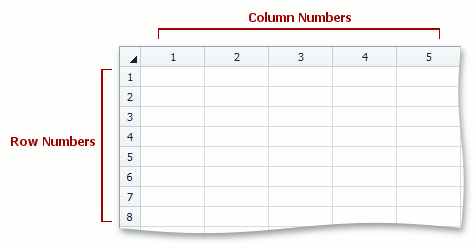A cell reference of the R1C1 style is a combination of “R” followed by the row number and “C” followed by the column number.

Cell Reference Description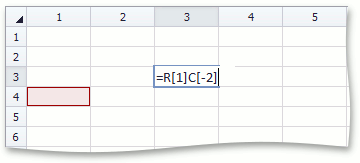A relative reference to the cell one row down and two columns to the left of the cell that contains the formula.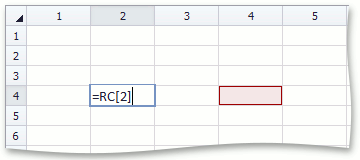A relative reference to the cell in the same row and two columns to the right of the cell that contains the formula.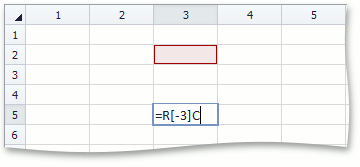A relative reference to the cell three rows up and in the same column of the cell that contains the formula.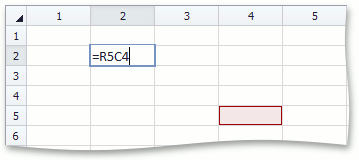An absolute reference to the cell in the fifth row and fourth column.

This example demonstrates how to create formulas using the R1C1 reference style. To do this, switch on the DocumentSettings.R1C1ReferenceStyle option and assign a formula string containing R1C1 cell references to the CellRange.Formula property. To obtain a cell reference in the R1C1 reference style, you can use the CellRange.GetReferenceR1C1 method.

``````// Switch on the R1C1 reference style in a workbook.
workbook.DocumentSettings.R1C1ReferenceStyle = true;

// Specify a formula with relative R1C1 references in cell D2
// to add values contained in cells A2 through A11.
worksheet.Cells["D2"].Formula = "=SUM(RC[-3]:RC[-3])";

// Specify a formula with absolute R1C1 references
// to add values contained in cells A2 through A11.
worksheet.Cells["D3"].Formula = "=SUM(R2C1:R11C1)";
``````